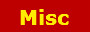##SAS example convert character to numericAsked by Anonymous - SAS example convert character to numeric: The following is a sas code example of how to convert a character alpha field that was read with input statement into a numeric field for use in calculations for example. DATA FILEIN;    INFILE SAMPLE1;    INPUT @25 charval   \$CHAR 8.    numval = charval * 1; RUN; In this example we read in charval which is an 8 byte alpha character field but we simply take it times 1 to make a new numeric variable named numval that can be used in calculations etc.

 Anonymous Says: Also if you want to convert char to numeric in your SAS code and also get the absolute value, you can use ABS function in same block of sas code. DATA FILEIN;    INFILE SAMPLE1;    INPUT @25 charval   \$CHAR 8.    numval = charval * 1;    numval = ABS(numval); RUN;

Type the code: